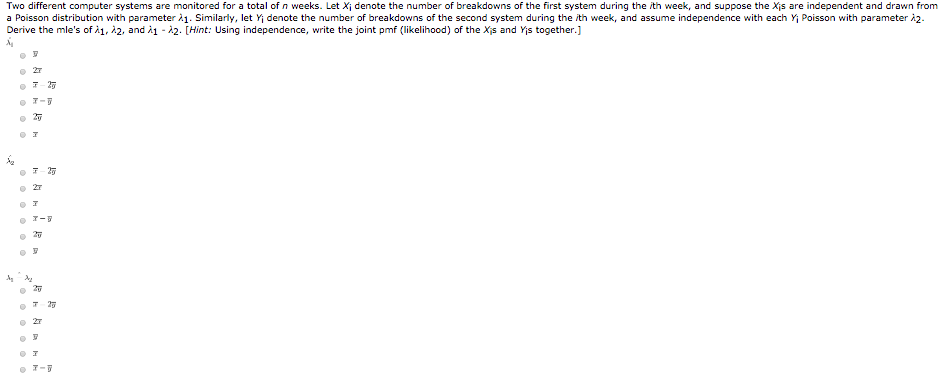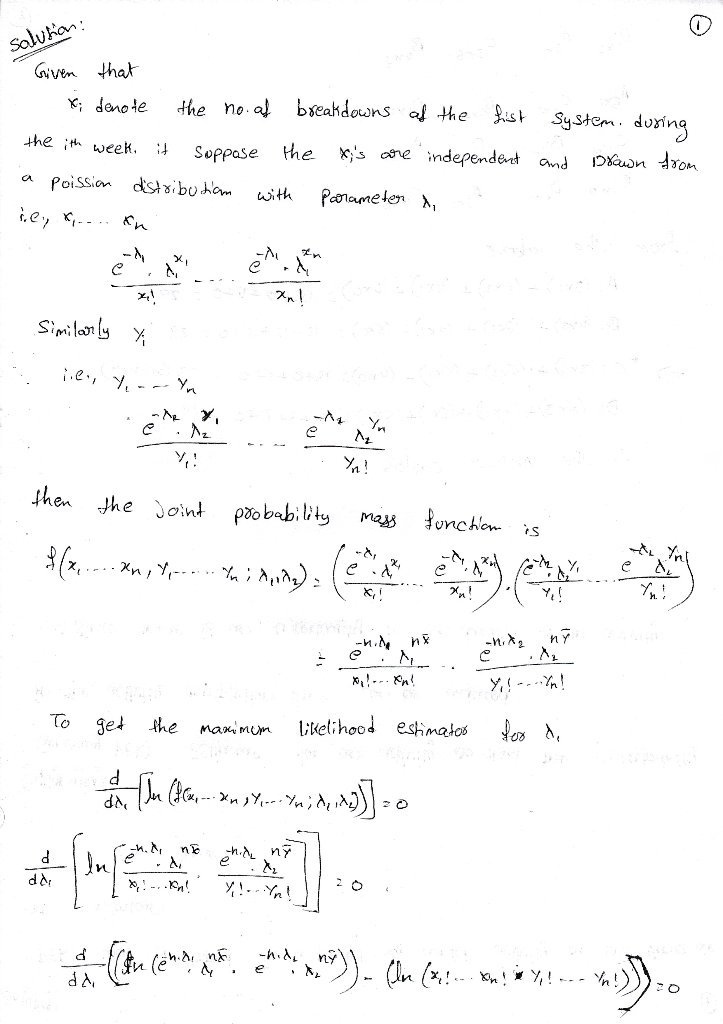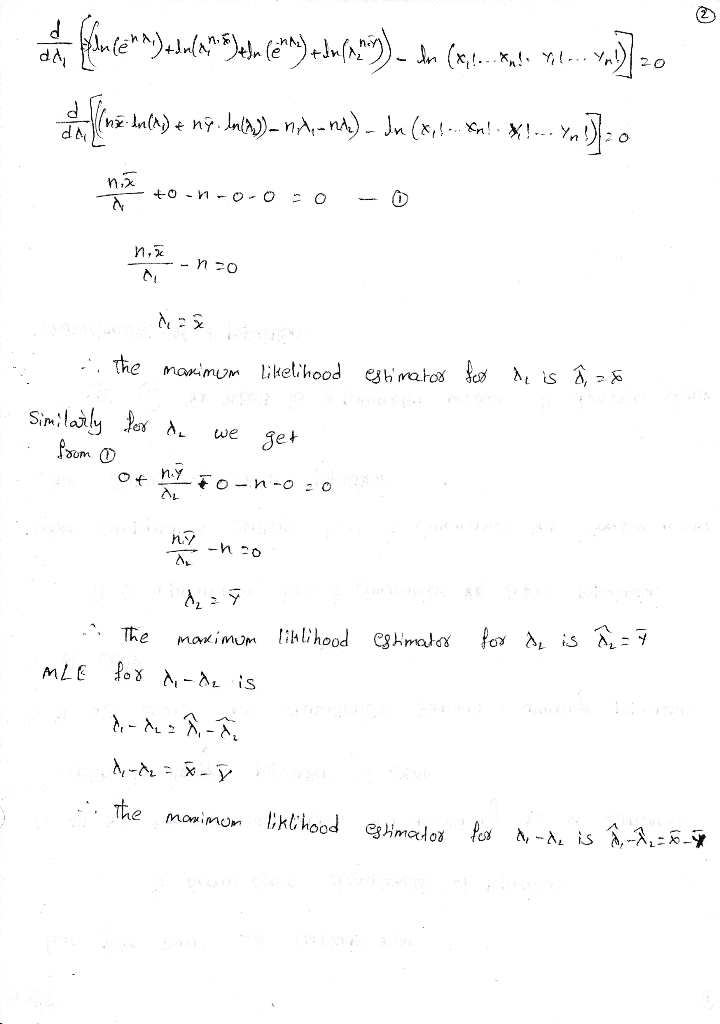# Two different computer systems are monitored for a total of n weeks. Let Xi denote the number of breakdowns of the firs...Two different computer systems are monitored for a total of n weeks. Let Xi denote the number of breakdowns of the first system during the ith week, and suppose the Xis are independent and drawn from a Poisson distribution with parameter A1. Similarly, let Yi denote the number of breakdowns of the second system during the ith week, and assume independence with each Yi Poisson with parameter i2. Derive the mle's of 1, A2, and i -2. [Hint: Using independence, write the joint pmf (likelihood) of the Xis and Yis together.] 2T T20 T-D T T- 7-7##### Add Answer of: Two different computer systems are monitored for a total of n weeks. Let Xi denote the number of breakdowns of the firs...
More Homework Help Questions Additional questions in this topic.